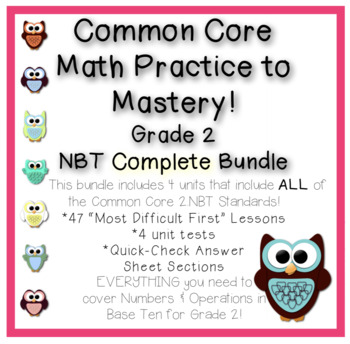# Common Core Math Practice to Mastery! Grade 2 NBT Complete Bundle2nd, Homeschool
Subjects
Standards
Resource Type
Formats Included
• PDF
Pages
128 pages

### Description

This 128 page bundle includes 4 "Math Practice to Mastery!" units that include ALL of the Common Core 2.NBT Standards!

*47 “Most Difficult First” Lessons

*4 unit tests

EVERYTHING you need to cover Numbers & Operations in Base Ten for Grade 2!

The Math Practice to Mastery! program utilizes the "Most Difficult First" method, where students who already have the skill mastered can become tutor leaders in your class and not have to do the repetitive practice that most learners need. This unique method gives students who need the repetition the opportunity for enough practice to master the skills. It's a Win-Win!

Did you know that one standard can have several skills involved? Standards 2.NBT.1, 2.NBT.2, 2.NBT.3 AND 2.NBT.4 involve 14 skills:

2.NBT.1.C1 (Concept 1) Place Values--2 digits

2.NBT.1.C2 (Concept 2) Place Values--3 digits

2.NBT.1.C3 (Concept 3) Place Values--3 digits, expanded form

2.NBT.1.C4 (Concept 4) Place Values--Logical thinking

2.NBT.2.C2 (Concept 2) Count forward by 1s within 1000

2.NBT.2.C1 (Concept 1) Count backward by 1s within 1000

2.NBT.2.C3 (Concept 3) Skip count by 100s within 1000

2.NBT.2.C4 (Concept 4) Skip count by 10s within 1000

2.NBT.2.C5 (Concept 5) Skip count by 5s within 1000

2.NBT.3.C1 (Concept 1) Convert from expanded form to 1000

2.NBT.3.C2 (Concept 2) Read number names to 1000

2.NBT.3.C3 (Concept 3) Write number names to 1000

2.NBT.4.C1 (Concept 1) Compare two 3-digit numbers

2.NBT.4.C2 (Concept2) Order three digit numbers

2.NBT.5 (Fluently add and subtract within 100 using strategies based on place value, properties of operations, and/or the relationship between addition and subtraction.) has 11 skills that must be mastered!

2.NBT.5.C1 (Concept 1) Addition: 2-digit + 1-digit

2.NBT.5.C2 (Concept 2) Addition: Balance 2-digit equations

2.NBT.5.C3 (Concept 3) Addition: Input/Output 2-digit tables

2.NBT.5.C4 (Concept 4) Addition: Two 2-digit numbers

2.NBT.5.C5 (Concept 5) Mixed Operations: 2-digit numbers

2.NBT.5.C6 (Concept 6) Mixed Operations: Balance 2-digit equations

2.NBT.5.C7 (Concept 7) Mixed Operations: Compare 2-digit equations

2.NBT.5.C8 (Concept 8) Subtraction: 2-digit Input/Output tables

2.NBT.5.C9 (Concept 9) Subtraction: 2-digit with 1-digit

2.NBT.5.C10 (Concept 10) Subtraction: Balance 2-digit equations

2.NBT.5.C11 (Concept 11) Subtraction: Two 2-digit numbers

Standards 2.NBT.6, 2.NBT.8 AND 2.NBT.9 have 9 skills that must be mastered!

I can: 1) add up to four two-digit numbers using strategies based on place value and properties of operations; 2) mentally add 10 or 100 to a given number 100–900, and mentally subtract 10 or 100 from a given number 100–900; and 3) explain why addition and subtraction strategies work, using place value and the properties of operations.)

2.NBT.6.C4 (Concept 4) Adding three 2-digit numbers

2.NBT.6.C2 (Concept 2) Adding four 2-digit numbers (multiples of 10)

2.NBT.6.C3 (Concept 3) Adding four 2-digit numbers (place value strategy)

2.NBT.6.C1 (Concept 1) Adding four 2-digit numbers

2.NBT.8.C1 (Concept 1) Mentally add 10 within 100-900

2.NBT.8.C2 (Concept 2) Mentally add 100 within 100-900

2.NBT.8.C3 (Concept 3) Mentally subtract 10 within 100-900

2.NBT.8.C4 (Concept 4) Mentally subtract 100 within 100-900

2.NBT.9.C1 (Concept 1) Adding two digit numbers using place values

2.NBT.7 (Fluently add and subtract within 1000 using strategies based on place value, properties of operations, and/or the relationship between addition and subtraction.) has 13 skills that must be mastered!

2.NBT.7.C1(Concept 1) Addition: 3-digit + 1-digit

2.NBT.7.C2(Concept 2) Addition: 3-digit + 2-digit

2.NBT.7.C3(Concept 3) Addition: Balance 3-digit equations

2.NBT.7.C4(Concept 4) Addition: Input/Output 3-digit tables

2.NBT.7.C5(Concept 5) Addition: 3-digit + 3-digit

2.NBT.7.C6(Concept 6) Mixed Operations: Two 3-digit numbers

2.NBT.7.C7(Concept 7) Mixed Operations: Balance 3-digit equations

2.NBT.7.C8(Concept 8) Mixed Operations: Compare 3-digit equations

2.NBT.7.C9(Concept 9) Subtraction: 3-digit Input/Output tables

2.NBT.7.C10(Concept 10) Subtraction: 3-digit minus 1-digit

2.NBT.7.C11(Concept 11) Subtraction: 3-digit minus 2-digit

2.NBT.7.C12(Concept 12) Subtraction: Balance 3-digit equations

2.NBT.7.C13(Concept 13) Subtraction: 3-digit minus 3-digit

My program includes significant practice and strategies to enable all students to master the skills! I'm beginning with 2nd grade and will continue development of the rest of the standards and other grade levels soon. Using this method, my second graders have gained up to 2 years in math skills in one semester!

Total Pages
128 pages
Included
Teaching Duration
1 Semester
Report this Resource to TpT
Reported resources will be reviewed by our team. Report this resource to let us know if this resource violates TpT’s content guidelines.

### Standards

to see state-specific standards (only available in the US).
Understand that the three digits of a three-digit number represent amounts of hundreds, tens, and ones; e.g., 706 equals 7 hundreds, 0 tens, and 6 ones. Understand the following as special cases:
100 can be thought of as a bundle of ten tens - called a “hundred.”
The numbers 100, 200, 300, 400, 500, 600, 700, 800, 900 refer to one, two, three, four, five, six, seven, eight, or nine hundreds (and 0 tens and 0 ones).
Count within 1000; skip-count by 5s, 10s, and 100s.
Read and write numbers to 1000 using base-ten numerals, number names, and expanded form.